Problem

# Given the circuit in Fig. 3.53, calculate the currents i1 through i4.Figure 3.53

Given the circuit in Fig. 3.53, calculate the currents i1 through i4.

Figure 3.53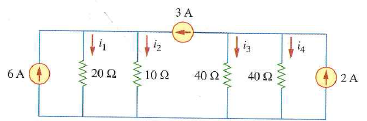#### Step-by-Step Solution

Solution 1

Redraw the circuit diagram as shown in Figure 1.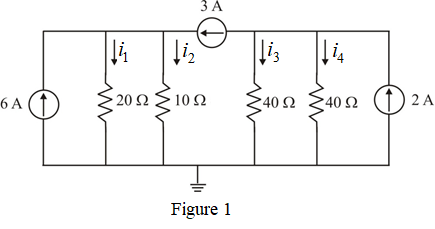The 20? and 10? resistors are in parallel. Their combined resistance is:The 40? and 40? resistors are in parallel. Their combined resistance is:The reduced circuit with nodes is shown in Figure 2.

Consider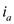be the current flowing through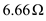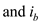be the current flowing through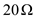resistor.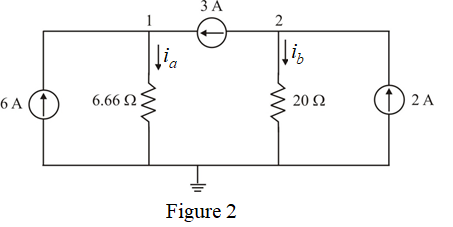Apply Kirchhoff's current law, the sum of currents entering the node is equal to the sum of the currents leaving from that node.

For the node 1, Kirchhoff's current law gives,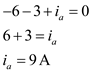Since, the Figure 2 is the modified circuit of Figure 1. Here, the resistoris the representation of two parallel connected resistors,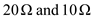.

This current,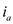flows through the 20? and 10? resistors in parallel as shown in Figure 3.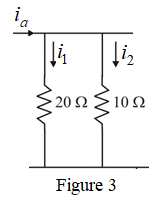Apply current division principle, to calculate the current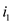.Therefore, the currentis,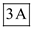.

Apply current division principle, to calculate the current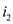.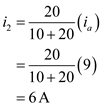Therefore, the currentis,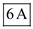.

Apply Kirchhoff's current law, the sum of currents entering the node is equal to the sum of the currents leaving from that node.

For the node 2, Kirchhoff's current law gives,Here, the resistor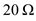is the representation of two parallel connected resistors,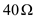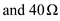.This current,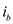flows through the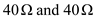resistors in parallel as shown in Figure 4.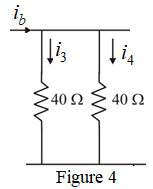Apply current division principle, to calculate the current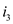.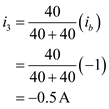Therefore, the currentis,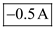.

Apply current division principle, to calculate the current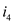.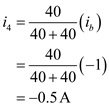Therefore, the currentis,.